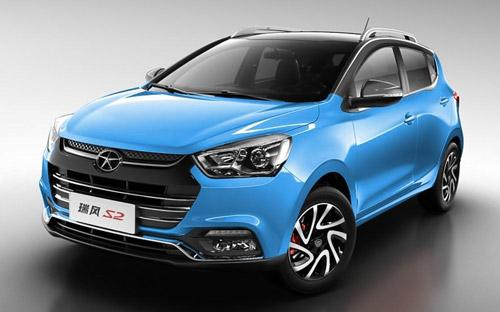江淮 瑞风S26 种颜色可选2016款最低售价：5.98 万元起

4135(mm)1750(mm)1568(mm)配置亮点：
• 胎压监测装置

• ISOFIX儿童座椅接口

• 车身稳定控制(ESC/ESP/DSC等)

• 电动天窗

• 定速巡航

• 后倒车雷达

• 真皮座椅

• GPS导航系统

• 氙气大灯

• 后视镜加热

2016款 1.5L CVT豪华智能型 双色版 (13张)
• 2016款 1.5L CVT豪华智能型 双色版 (13张)
• 2016款 1.5L 手动豪华智能型 双色版 (93张)江淮 瑞风S2 在售车型

排量 车型 厂商指导价 本地最低报价 购车工具
1.5L 1.5L 手动舒适型 双色版 5挡手动 5.98万 询底价+对比
1.5L 手动豪华型 双色版 5挡手动 6.38万 询底价+对比
1.5L 手动豪华智能型 双色版 5挡手动 6.88万 询底价+对比
1.5L CVT豪华型 双色版 CVT无级变速 7.18万 询底价+对比
1.5L CVT豪华智能型 双色版 CVT无级变速 7.68万 询底价+对比

江淮 瑞风S2 经销商

查看更多 >>
更多 >>

本地降价排名

江淮 瑞风S2 动力加速

瑞风S2 0-100公里加速时间分布在 0.0-秒 属于 超跑级

动力级别 加速时间 车型

江淮 瑞风S2 视频

江淮 瑞风S2 新闻资讯

抢先测试江淮瑞风S2手动/自动版

评测 超过11576次关注

瑞风S2动力系统匹配成熟，驾驭轻松，整体调校达到了很高的水准。像发动机噪音大、变速箱齿比太密不利于燃油经济性这样的问题也是存在的。

售5.88-7.58万元 江淮瑞风S2今日正式上市

新闻 超过8540次关注

8月31日，江淮旗下全新小型SUV瑞风S2正式上市，新车共推出五款车型，价格区间为5.88-7.58万元。

瑞风S2预售5.98万-7.68万 8月31日上市

新闻 超过8446次关注

江淮即将推出一款全新小型SUV，命名为“瑞风S2”。新车拟定于8月31日正式上市，售价或在6-8万元之间。如今这款新车已有清晰官图和配置曝光。

8月31日上市 江淮瑞风S2官图/配置曝光

新闻 超过9701次关注

江淮即将推出一款全新小型SUV，命名为“瑞风S2”。新车拟定于8月31日正式上市，售价或在6-8万元之间。如今这款新车已有清晰官图和配置曝光。

全新瑞风S2将于8月31日上市 搭1.5L动力

新闻 超过8596次关注

江淮全新小型SUV车型瑞风S2已经在2015上海车展正式亮相，据国内媒体最新消息，新车将在8月31日正式上市，售价或在6-8万元之间。

瑞风S2汽油版8月上市 电动版年底推出

新闻 超过7777次关注

江淮全新小型SUV车型瑞风S2已经在2015上海车展正式亮相，新车将在8月份上市。另外，瑞风S2电动版车型则将会在年底发布，预计是在11月的广州车展。

猜你喜欢

﻿
• 快速找车
• 选择品牌
• 选择品牌
• A  奥迪
• A  阿斯顿·马丁
• A  阿尔法·罗密欧
• B  宝沃
• B  布加迪
• B  巴博斯
• B  保时捷
• B  宾利
• B  奔驰
• B  宝马
• B  本田
• B  别克
• B  标致
• B  比亚迪
• B  宝骏
• B  北汽制造
• B  北汽新能源
• B  北汽幻速
• B  北汽威旺
• B  北京汽车
• B  奔腾
• B  北汽绅宝
• C  长安
• C  长安商用
• C  长城
• C  昌河
• D  大众
• D  道奇
• D  DS
• D  东南
• D  东风风神
• D  东风风行
• D  东风小康
• D  东风风度
• D  东风
• F  福特
• F  丰田
• F  菲亚特
• F  法拉利
• F  福田
• F  福迪
• F  福汽启腾
• G  观致
• G  广汽传祺
• G  广汽吉奥
• G  GMC
• H  红旗
• H  汉腾汽车
• H  哈弗
• H  哈飞
• H  海格
• H  海马
• H  华颂
• H  黄海
• H  华泰
• H  恒天
• J  吉利汽车
• J  捷豹
• J  Jeep
• J  江淮
• J  江铃
• J  金杯
• J  九龙
• J  金旅
• K  凯翼
• K  凯迪拉克
• K  克莱斯勒
• K  科尼塞克
• K  卡威
• K  开瑞
• L  路虎
• L  林肯
• L  劳斯莱斯
• L  兰博基尼
• L  雷克萨斯
• L  铃木
• L  雷诺
• L  理念
• L  力帆
• L  莲花汽车
• L  猎豹
• L  路特斯
• L  陆风
• M  马自达
• M  MG
• M  MINI
• M  玛莎拉蒂
• M  摩根
• M  迈凯轮
• N  纳智捷
• O  欧宝
• O  讴歌
• O  欧朗
• Q  奇瑞
• Q  起亚
• Q  启辰
• R  日产
• R  荣威
• R  瑞麒
• S  三菱
• S  斯威汽车
• S  萨博
• S  smart
• S  斯柯达
• S  斯巴鲁
• S  思铭
• S  双龙
• S  上汽大通
• S  双环
• T  特斯拉
• T  腾势
• W  沃尔沃
• W  五菱汽车
• W  五十铃
• W  威兹曼
• W  威麟
• X  现代
• X  雪佛兰
• X  雪铁龙
• X  西雅特
• Y  一汽
• Y  英菲尼迪
• Y  英致
• Y  依维柯
• Y  野马汽车
• Y  永源
• Z  众泰
• Z  中华
• Z  中兴
• Z  知豆
• 选择车系
• 选择车系
• 车型对比
• 选择品牌
• 选择品牌
• A  奥迪
• A  阿斯顿·马丁
• A  阿尔法·罗密欧
• B  宝沃
• B  布加迪
• B  巴博斯
• B  保时捷
• B  宾利
• B  奔驰
• B  宝马
• B  本田
• B  别克
• B  标致
• B  比亚迪
• B  宝骏
• B  北汽制造
• B  北汽新能源
• B  北汽幻速
• B  北汽威旺
• B  北京汽车
• B  奔腾
• B  北汽绅宝
• C  长安
• C  长安商用
• C  长城
• C  昌河
• D  大众
• D  道奇
• D  DS
• D  东南
• D  东风风神
• D  东风风行
• D  东风小康
• D  东风风度
• D  东风
• F  福特
• F  丰田
• F  菲亚特
• F  法拉利
• F  福田
• F  福迪
• F  福汽启腾
• G  观致
• G  广汽传祺
• G  广汽吉奥
• G  GMC
• H  红旗
• H  汉腾汽车
• H  哈弗
• H  哈飞
• H  海格
• H  海马
• H  华颂
• H  黄海
• H  华泰
• H  恒天
• J  吉利汽车
• J  捷豹
• J  Jeep
• J  江淮
• J  江铃
• J  金杯
• J  九龙
• J  金旅
• K  凯翼
• K  凯迪拉克
• K  克莱斯勒
• K  科尼塞克
• K  卡威
• K  开瑞
• L  路虎
• L  林肯
• L  劳斯莱斯
• L  兰博基尼
• L  雷克萨斯
• L  铃木
• L  雷诺
• L  理念
• L  力帆
• L  莲花汽车
• L  猎豹
• L  路特斯
• L  陆风
• M  马自达
• M  MG
• M  MINI
• M  玛莎拉蒂
• M  摩根
• M  迈凯轮
• N  纳智捷
• O  欧宝
• O  讴歌
• O  欧朗
• Q  奇瑞
• Q  起亚
• Q  启辰
• R  日产
• R  荣威
• R  瑞麒
• S  三菱
• S  斯威汽车
• S  萨博
• S  smart
• S  斯柯达
• S  斯巴鲁
• S  思铭
• S  双龙
• S  上汽大通
• S  双环
• T  特斯拉
• T  腾势
• W  沃尔沃
• W  五菱汽车
• W  五十铃
• W  威兹曼
• W  威麟
• X  现代
• X  雪佛兰
• X  雪铁龙
• X  西雅特
• Y  一汽
• Y  英菲尼迪
• Y  英致
• Y  依维柯
• Y  野马汽车
• Y  永源
• Z  众泰
• Z  中华
• Z  中兴
• Z  知豆
• 选择车系
• 选择车系
• 选择车型
• 选择车型
• 意见反馈